Algorithm - Interview Questions and Answers for 'Graph traversal' | Search Java Interview Question - javasearch.buggybread.com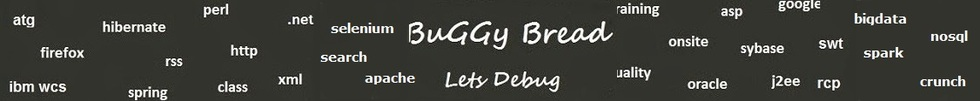# Search Interview QuestionsMore than 3000 questions in repository.There are more than 900 unanswered questions.Have a video suggestion.
Click Correct / Improve and please let us know.
Label / Company      Label / Company / TextSubmit Question

Algorithm - Interview Questions and Answers for 'Graph traversal' - 7 question(s) found - Order By NewestQ1. What is the difference between Graph's Breadth first and Depth First algorithm ?Algorithm2017-07-23 10:12:58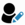Ans. In Breadth first algorithm, all the adjacent nodes of the starting node is visited first and then the same rule is followed while moving inwards whereas

In Depth first algorithm, all the nodes of a single traversal path are visited first till a cycle or an end is found.

For example , given the following entries of adjacent nodes

1,2
1,3
1,6
2,4
2,5
3,6

The Breadth first path would be

1,2,3,6,4,5

and Depth first path would be

1,2,4,5,3,6Help us improve. Please let us know the company, where you were asked this question :LikeDiscussCorrect / Improvegraph traversal  breadth first vs depth first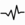frequentQ2. Write a Program for Graph Depth First Traversal using Apache Commons MultiMapAlgorithm2017-07-23 10:01:14Ans. import java.util.ArrayList;
import java.util.Collection;
import java.util.HashSet;
import java.util.Set;

public class Graph {
private static Multimap<Integer,Integer> adjacentDirectedNodesMap = ArrayListMultimap.create();
private static Set<Integer> alreadyVisited = new HashSet();

static{
}

public static void main(String[] args){
ArrayList visited = new ArrayList();

Integer startNode = 1;

}

return;
}
System.out.println(integer);
}
}

}Help us improve. Please let us know the company, where you were asked this question :LikeDiscussCorrect / Improvegraph traversal  depth first algorithmQ3. Write a Program for Graph Breadth First Traversal using Apache Commons MultiMap2017-07-23 10:07:15Ans. import java.util.ArrayList;
import java.util.Collection;
import java.util.HashSet;
import java.util.Map;
import java.util.Set;

public class Graph {
private static Multimap<Integer,Integer> adjacentDirectedNodesMap = ArrayListMultimap.create();
private static Set<Integer> alreadyVisited = new HashSet();

static{
}

public static void main(String[] args){
ArrayList visited = new ArrayList();

Integer startNode = 1;

}

System.out.println(integer);
continue;
}
System.out.println(integer1);
}
}
}

}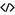Sample Code for import java.util.ArrayList; import java.util.Collection; import java.util.HashSet; import java.util.Map; import java.util.Set; import com.google.common.collect.ArrayListMultimap; import com.google.common.collect.Multimap;Help us improve. Please let us know the company, where you were asked this question :LikeDiscussCorrect / Improvegraph traversal  breadth first traversalQ4. Write an Algorithm for Graph Traversal ? The Graph has a loop.Algorithm2016-11-30 15:42:32Ans. Please not that all such questions can be easily answered through recursion.

Simple recursive implementation could be

traverse(root);

void traverse(Element element){
if(element.hasNext()){
traverse(element.next());
} else {
System.out.println(element);
}
}

but this algo / code lead to endless loop if there is a loop in graph traversal.

So you can keep a collection to keep track of which elements have laready been traversed

static List<Elements> listOfAlreadyTraversedElements = new ArrayList<Elements>();

main(){
traverse(root);
}

void traverse(Element element){
if(element.hasNext()){
traverse(element.next());
} else {
System.out.println(element);
}
}Help us improve. Please let us know the company, where you were asked this question :LikeDiscussCorrect / Improvegraph traversal algorithm  graph traversal algorithm using recursion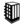Asked in 1 Companies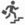intermediateQ5. Given the list of adjacent nodes, What will be the Breadth First path

1 -> 2
1 -> 3
1 -> 6
2 -> 4
3 -> 5

Algorithm2017-07-23 10:30:20Ans. 1 -> 2 -> 3 -> 6 -> 4 - > 5Help us improve. Please let us know the company, where you were asked this question :LikeDiscussCorrect / Improvebreadth first traversal  graph traversalQ6. Given the list of adjacent nodes, What will be the Depth First path

1 -> 2
1 -> 3
1 -> 6
2 -> 4
3 -> 5
Algorithm2017-07-23 10:30:48Ans. 1 -> 2 -> 4 -> 3 -> 5 -> 6Help us improve. Please let us know the company, where you were asked this question :LikeDiscussCorrect / Improvedepth first traversal  graph traversalQ7. What will happen in a graph traversal if we don't have a check for cycleAlgorithm2017-07-23 10:32:30Ans. It will result in never ending loopHelp us improve. Please let us know the company, where you were asked this question :LikeDiscussCorrect / Improvegraph traversal

## Help us and Others Improve. Please let us know the questions asked in any of your previous interview.

Any input from you will be highly appreciated and It will unlock the application for 10 more requests.